## Excel DDB Function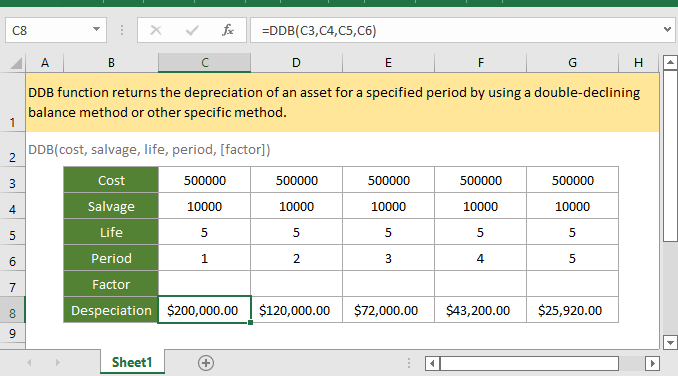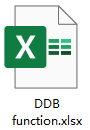### Description

The DDB function returns the depreciation of an asset for a specified period by using a double-declining balance method or other specific method.

### Syntax and arguments

Formula syntax

DB(cost, salvage, life, period, [factor])

Arguments

 Cost: Required, the initial cost of the asset. Salvage: Required, the value of the asset at the end of the depreciation, or called the salvage value of the asset. Life: Required, the number of periods over which the asset will be depreciation, or the useful life of the asset. Period: Required, the period which used to calculate the depreciation for. Factor: Optional, the rate that used in the depreciation calculation. If it is omitted, the DDB function assumes it as 2.

Return Value

The DDB function returns a numeric value in currency format.

Errors

1. If the arguments are non-numeric value, the function returns #VALUE! error value.

2. If anyone of below occurs, the function returns ##NUM! error value:

• Cost < 0, or salvage < 0;
• Life or period <= 0;
• Factor <=0;
• Period > life.

Remarks

1. Both of the life and period must be in the same unit. For instance, the life is calculated in yearly, the period must be calculated in yearly too.

2. The double-declining balance method computes the depreciation of asset at an accelerated rate. In the first period, depreciation is the highest, and then decreases in following periods. The DDB function use below formula to calculate the depreciation for a period:

Min( (cost - total depreciation from prior periods) * (factor/life), (cost - salvage - total depreciation from prior periods) )

Factor can be changed if you do not want to use the double-declining balance method for depreciation calculation.

Use the VDB function if you want to switch to the straight-line depreciation method when depreciation is greater than the declining balance calculation.

Version

Excel 2003 or later

### Usage and Examples

Example 1 when argument factor is omitted

Supposing in the table B3:G8 which lists the cost, salvage, life and periods (1-5), and factors are omitted (will take it as 2), to calculate the depreciation of the asset in each period, please use below formula:

=DDB(C3,C4,C5,C6))

Press Enter key to get the depreciation of first period.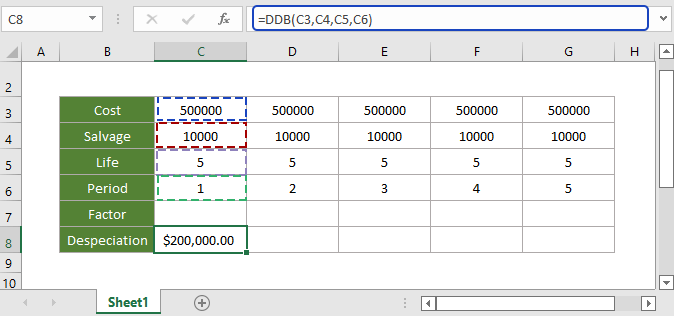Then select first result, drag autofill handle over the cells to calculate the depreciations in other periods.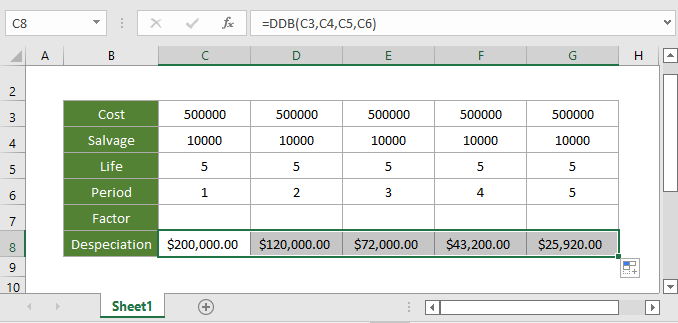Example 2 when argument factor is other number

Supposing in the table B3:G8 which lists the cost, salvage, life and periods (1-5), and the factor is 3, to calculate the depreciation of the asset in each period, please use below formula:

=DDB(C3,C4,C5,C6,C7)

Press Enter key to get the depreciation of first period.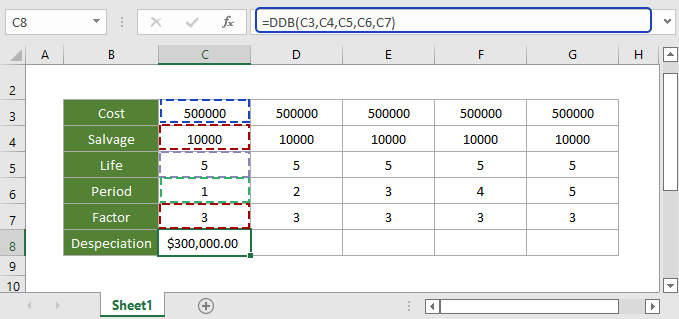Then select first result, drag autofill handle over the cells to calculate the depreciations in other periods.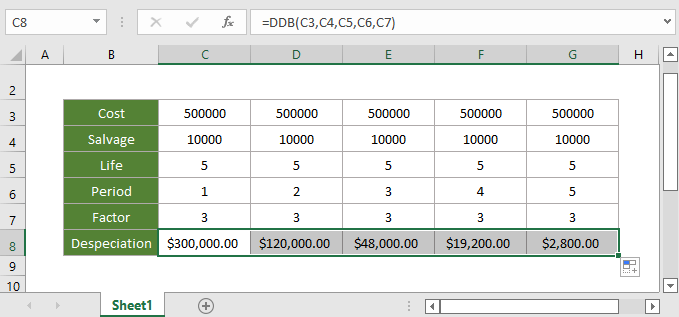#### Relative Functions:

• Excel CUMIPMT Function
The CUMIPMT function returns the cumulative interest paid on a load between the start period and end period.

• Excel DB Function
The DB function returns the depreciation of an asset for a specified period by using a fixed-declining balance method.

• Excel ACCRINTM Function
The ACCRINTM function returns the accrued interest for a security that pays interest at maturity.

• Excel AMORDEGRC Function
The AMORDEGRC function returns the linear depreciation of an asset for each accounting period by applying a depreciation coefficient based on the lifetime of the assets.

### The Best Office Productivity Tools

#### Kutools for Excel - Helps You To Stand Out From Crowd

 Popular Features: Find, Highlight or Identify Duplicates  |  Delete Blank Rows  |  Combine Columns or Cells without Losing Data  |  Round without Formula ... Super VLookup: Multiple Criteria  |  Multiple Value  |  Across Multi-Sheets  |  Fuzzy Lookup... Adv. Drop-down List: Easy Drop Down List  |  Dependent Drop Down List  |  Multi-select Drop Down List... Column Manager: Add a Specific Number of Columns  |  Move Columns  |  Toggle Visibility Status of Hidden Columns  |  Compare Columns to Select Same & Different Cells ... Featured Features: Grid Focus  |  Design View  |  Big Formula Bar  |  Workbook & Sheet Manager | Resource Library (Auto Text)  |  Date Picker  |  Combine Worksheets  |  Encrypt/Decrypt Cells  |  Send Emails by List  |  Super Filter  |  Special Filter (filter bold/italic/strikethrough...) ... Top 15 Toolsets:  12 Text Tools (Add Text, Remove Characters ...)  |  50+ Chart Types (Gantt Chart ...)  |  40+ Practical Formulas (Calculate age based on birthday ...)  |  19 Insertion Tools (Insert QR Code, Insert Picture from Path ...)  |  12 Conversion Tools (Numbers to Words, Currency Conversion ...)  |  7 Merge & Split Tools (Advanced Combine Rows, Split Excel Cells ...)  |  ... and more

Kutools for Excel Boasts Over 300 Features, Ensuring That What You Need is Just A Click Away...#### Office Tab - Enable Tabbed Reading and Editing in Microsoft Office (include Excel)

• One second to switch between dozens of open documents!
• Reduce hundreds of mouse clicks for you every day, say goodbye to mouse hand.
• Increases your productivity by 50% when viewing and editing multiple documents.
• Brings Efficient Tabs to Office (include Excel), Just Like Chrome, Edge and Firefox.